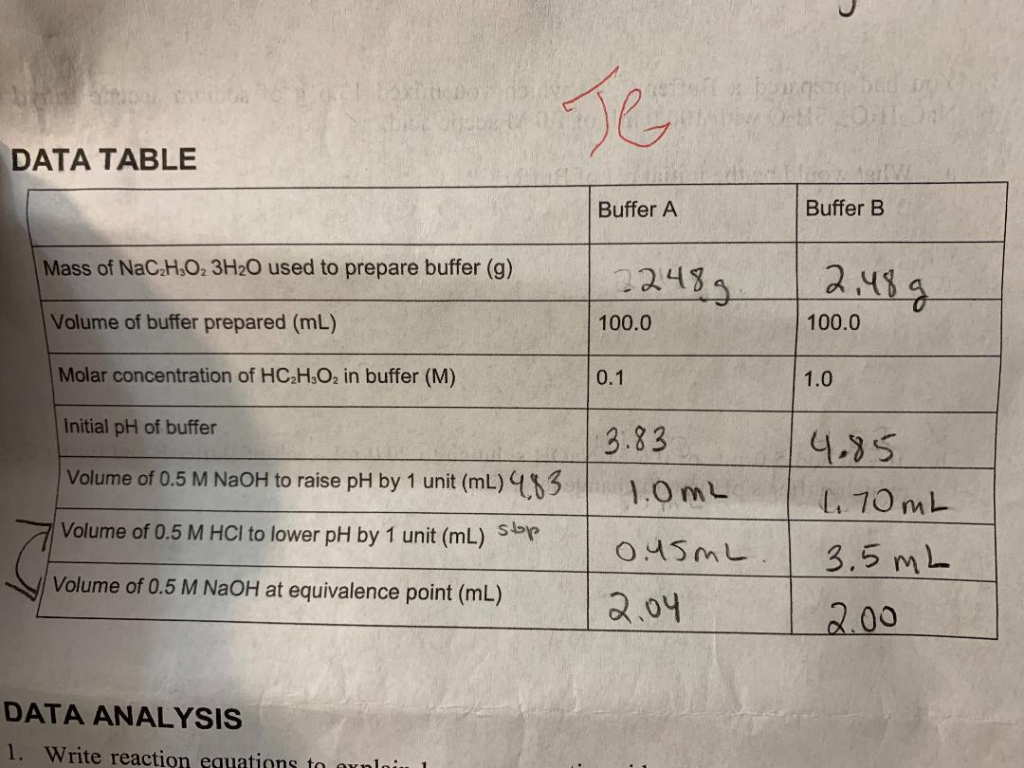Homework Help Question & Answers

# Hi there, I totally understand what is happening and what they are asking but I cannot get passed...

Hi there,

I totally understand what is happening and what they are asking but I cannot get passed the math.

Can someone please break this question down for me? also the data in the last two rows is in ML. Please specify what you mean by unclear?

Buffer capacity has a rather loose definition, yet it is an important property of buffers. A commonly seen definition of buffer capacity is: “the amount of H+ or OH– that can be neutralized before the pH changes to a significant degree.” Use your data to determine the buffer capacity of Buffer A and Buffer B.

Thank you!DATA TABLE Buffer A Buffer B Mass of NaC2H02 3H20 used to prepare buffer (g) 2243 100.0 2.4s a Volume of buffer prepared (mL) 100.0 Molar concentration of HC.H O2 in buffer (M) Initial pH of buffer Volume of 0.5 M NaOH to raise pH by 1 unit (mL) qN3 Volume of 0.5 M HCI to lower pH by 1 unit (mL) Sbrp Volume of 0.5 M NaOH at equivalence point (mL) 0.1 1.0 3.83 | .35 .0 mL 045m | 2.04 3.5 mL DATA ANALYSIS 1. Write reaction equations to arnl

#### Homework Answers

ReportAnswer #1

Definition of buffer capacity: Buffer capacity is defined as the amount of strong acid or strong base added to 1L of a buffer to change the pH of the buffer by 1.0 unit.

The formula is:

Buffer capacity = (moles of H+ or OH- added) / [(change in pH)*(volume of buffer in L)

Buffer capacity of a solution may have different value for strong acid or strong base.

Buffer-A:

Buffer capacity for strong base NaOH

Moles of OH- or NaOH added = 0.5 mol/L * 0.001 L = 0.0005 mol

Volume of buffer = 100 mL * (1L / 1000 mL) = 0.100 L

Change in pH = 1.0

=> Buffer capacity = 0.0005/ (1 * 0.100) = 0.005 (Answer)

Buffer capacity for strong acid HCl:

Moles of H+ or HCl added = 0.5 mol/L * 0.00045 L = 0.000225 mol

Volume of buffer = 100 mL * (1L / 1000 mL) = 0.100 L

Change in pH = 1.0

=> Buffer capacity = 0.000225/ (1 * 0.100) = 0.00225 (Answer)

Buffer-B:

Buffer capacity for strong base NaOH

Moles of OH- or NaOH added = 0.5 mol/L * 0.0017 L = 0.00085 mol

Volume of buffer = 100 mL * (1L / 1000 mL) = 0.100 L

Change in pH = 1.0

=> Buffer capacity = 0.00085/ (1 * 0.100) = 0.0085 (Answer)

Buffer capacity for strong acid HCl:

Moles of H+ or HCl added = 0.5 mol/L * 0.0035 L = 0.00175 mol

Volume of buffer = 100 mL * (1L / 1000 mL) = 0.100 L

Change in pH = 1.0

=> Buffer capacity = 0.00175/ (1 * 0.100) = 0.0175 (Answer)

Add Homework Help Answer
##### Add Answer of: Hi there, I totally understand what is happening and what they are asking but I cannot get passed...
Your Answer: Your Name: What's your source?
Not the answer you're looking for? Ask your own homework help question. Our experts will answer your question WITHIN MINUTES for Free.
More Homework Help Questions Additional questions in this topic.

• #### of 250 candidates writing a exam, 147 passed in math,150 pass in chemistry and 85 pass subjects B)calculate the number of students who pass i)pass math only ii)pass chemistry only iii)passed neither iv)pass only one subject

Need Online Homework Help?

Get FREE EXPERT Answers
WITHIN MINUTES
Related Questions